# Can a computer learn to detect anime characters?

Posted on June 7, 2014

As I explained in my previous post, I’m trying to automatically identify the anime characters in an anime image. As a first step, I’d like to eliminate the irrelevant information contained in the background of the image. My first approach combined GrabCut, which attempts to model the background of the image given minimal user input, and saliency detection which I hoped would be able to pick up the characters in the image.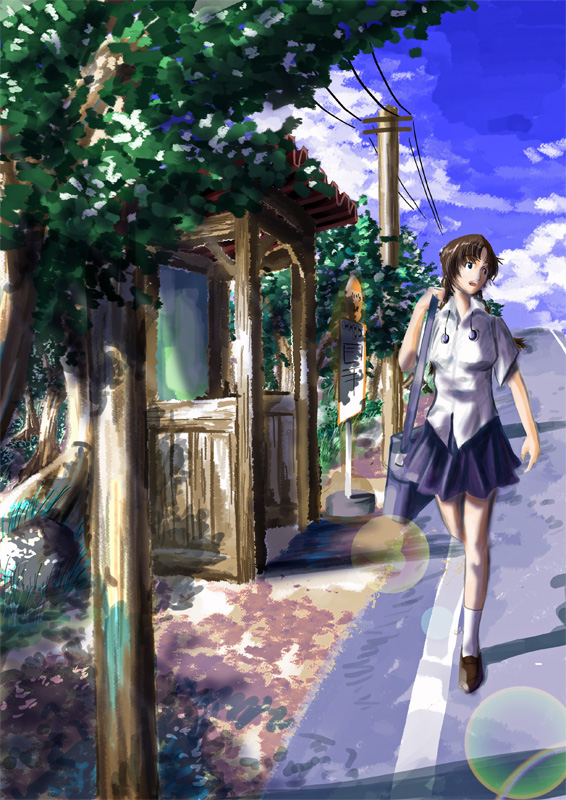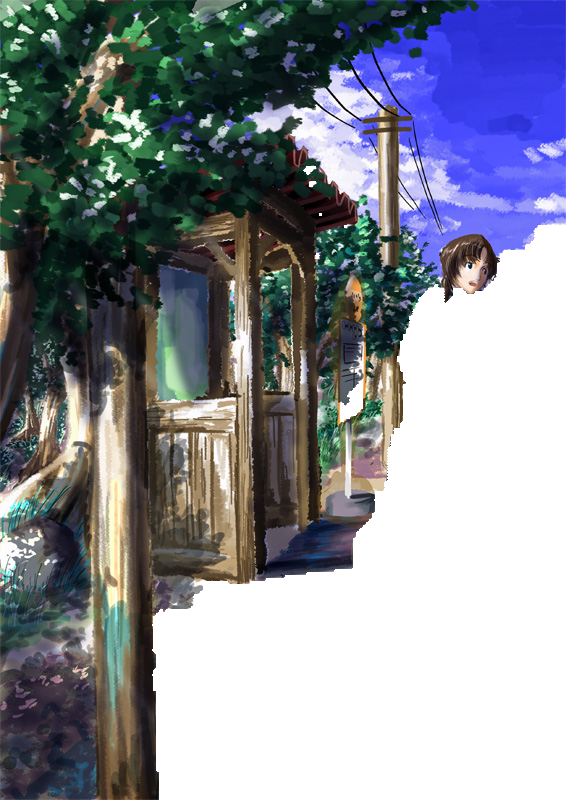Here the character is drawn in the same style as the background, and saliency detection fails to pick it up. Hence the decapitated schoolgirl.

After running some benchmarks, it turns out that saliency detection is quite inefficient for finding the location of the character in the image. Simply using a Gaussian map centered on the image gives better results. This is due to the fact that saliency detection doesn’t know what a character is - it justs identifies areas of the images which stand out to a human observer. So we would like an algorithm smart enough to know what a character looks like, and use that information to detect it.

There are many object detection which can, given enough examples of an object, effectively detect it in an image. A couple of them stand out from the rest in terms of efficiency. Viola and Jones’s object detection algorithm, although it dates from 2001, is still very much used thanks to overall computational efficiency and detection performance, with high quality implementations freely available. While it excels at detecting relatively static patterns like people’s face in a photo, it has more trouble with deformable objects. Felzenszwalb and Girschick’s method successfully solves this issue by explicitely modeling the parts of the object to detect, as well as its deformations - although implementations are still lacking. As anime character images are characterized by large variations in posture and drawing style, I chose to study this algorithm for anime images.

## Learning a model for anime characters

To learn what an anime character looks like, Felzenszwalb’s method requires many, many examples of characters. For that purpose, I scraped a thousand anime images from deviantArt and manually put a bounding box around the characters so the algorithm knows where to look. The algorithm also needs example of what an anime character doesn’t look like, which is more complicated to obtain. Indeed, while it is easy in the case of natural images to just take a photo with no objects of interest, very few people draw anime style artwork without characters in them. So I decided to automatically generate them by cutting pieces from the background of the character images.

I mentioned that this method models the parts of the objects automatically. The awesome thing is, it doesn’t require any examples for that - just give the algorithm a bounding box of the objects, and it will be able to find the right parts for detection. To see what kind of parts it infers, I trained the algorithm on the entire dataset of a thousand images as a first step.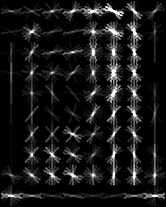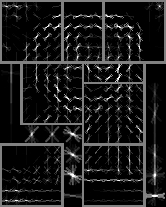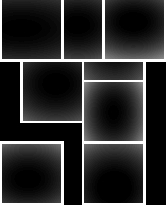Model infered by the algorithm on a large dataset of images. First image: the root filter which specifies the global shape of the object to detect. Second image: the parts filter, rectangular boxes which specify the shape of individual parts of the object, at a finer level of detail. Last image: deformation costs for the parts. Each rectangle specifies a deformation penalty for the corresponding part. Black means no penalty, and white means high penalty. Squinting a little, one might distinguish the shape of the head and chest of a character.

The model seems to pick up on the face and chest area of a character. This gives some confidence that training resulted in a meaningful model. To verify this intuition, I would like to measure how well it would perform against data it hasn’t encountered before.

For this purpose I employed cross validation, randomly splitting the 1000 images dataset into five 200 images datasets. Each of them is used for testing the model trained on the 800 other images. Looking at how consistent the results are across the 5 datasets, we can get a sense of the way the algorithm deals with unseen data. If we get similar (hopefully good) results for each dataset, then the method probably managed to avoid overfitting to its training set. Otherwise, we’re in trouble.

## Evaluating character detections

Of course, this presumes a way to measure the ability of the trained model to correctly detect characters in the images of the dataset. For a given image, the algorithm gives us a set of detected bounding boxes $$D = \{D_1, ..., D_n\}$$ where it finds characters. If we also have a set of ground truth bounding boxes $$G = \{G_1, ..., G_m\}$$ specifying where the characters actually are in the image, we can compare the two and deduce a quality measure for the detection.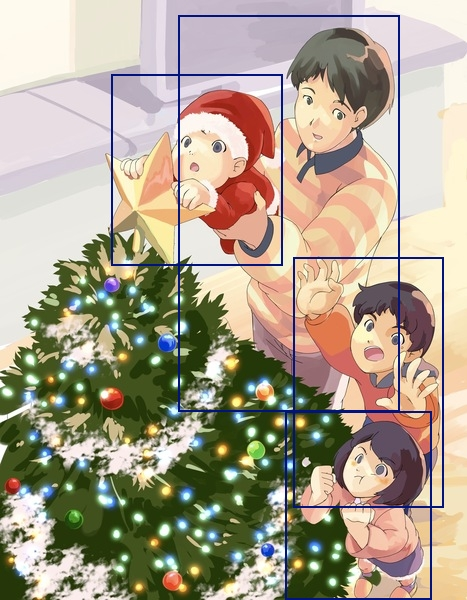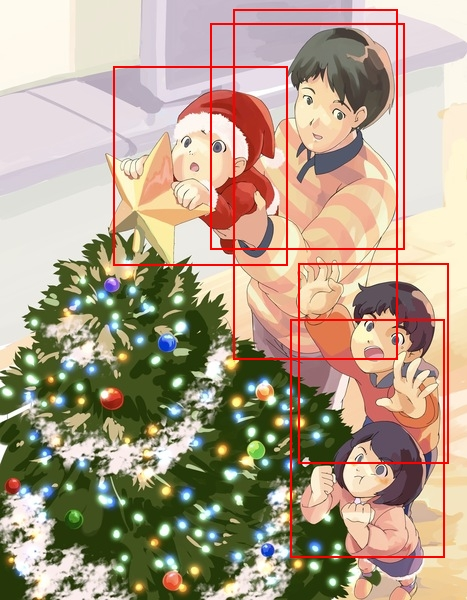Example of an image with ground truth bounding boxes (blue), and detected bounding boxes (red).

A simple starting point is to consider the character correctly detected when its ground truth box $$G_i$$ overlaps a detected box $$D_j$$ for at least 50%, i.e. using set theoretical notation:

$\frac{|G_i \cap D_j|}{|G_i \cup D_j|} \geq \frac{1}{2}$

However, this does not tell us how to deal with multiple detections - e.g. when multiple detected boxes overlap a single ground truth box for more than 50%. Nor does it tell us what to do with ambiguous detection, where multiple ground truth boxes overlap a single detected box - which character should be considered detected? It turns out to be a difficult issue to solve. The PASCAL object detection competition and dataset takes the point of view that multiple detections should be penalized: only one detection is considered correct, and all the other are considered false positives - regardless of whether or not they would correctly detect another object in the image. Ambiguous detection is not mentioned, presumably because the PASCAL dataset does not include overlapping ground truth boxes for a given object class. However, in our case it is common to have such overlapping boxes, so we have to deal with that too. Furthermore, multiple detections are not necessarily a bad thing in the context of a web artist community, and should not be overly penalized.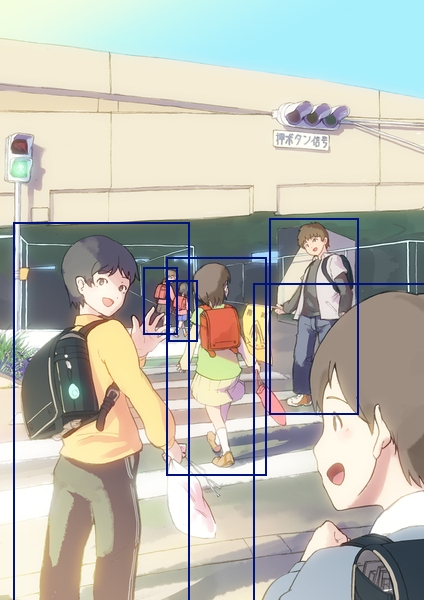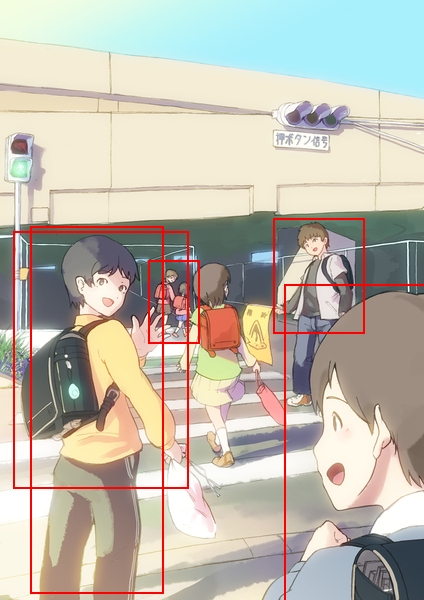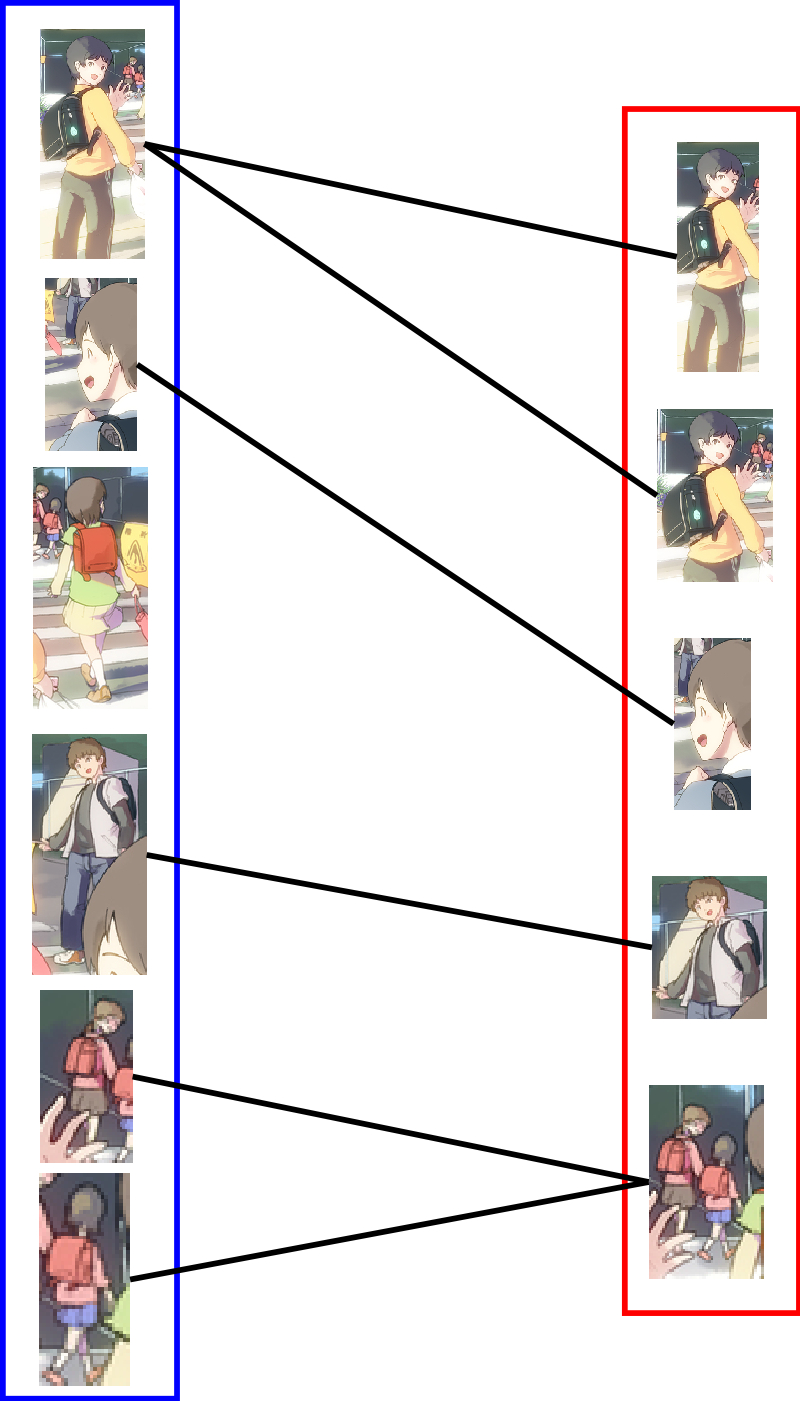Image with ground truth boxes (blue), detected boxes (red), and corresponding overlap graph $$B$$ with set $$G$$ of ground truths on the left and $$D$$ of detections on the right. We have one case of multiple detection (the boy on the left) and one case of ambiguous detection (the two children in the background).

I quickly realized that thinking about the issue in terms of the boxes is counter productive, as we really only care about the overlappings between them. Modeling these overlappings using a graph where vertices are boxes and edges are overlappings therefore makes a lot of sense. Consider the bipartite graph $$B = (V = (G, D), E)$$ where $$G$$ is the set of ground truth boxes, and $$D$$ the set of detected boxes, with an edge between boxes $$G_i$$ and $$D_j$$ if and only if they overlap more than 50%. It is then easy to visualize that multiple detections correspond to vertices in $$G$$ with more than one edge, and ambiguous detections correspond to vertices in $$D$$ with more than one edge. So we would like to find a subgraph $$S$$ of $$B$$ where all such nodes have exactly one edge between them, solving both cases of multiple and ambiguous detections.

It turns out that this is a well studied problem in graph theory called the maximum matching problem, for which efficient algorithms have been devised. This also tells us that there is not always only one such matching between ground truth and detected boxes, and so $$S$$ is not unique. However, this is not an issue as we only care about the number of edges in $$S$$ which is the same no matter which one we choose - otherwise it wouldn’t be a maximum matching by definition. Indeed, the number of edges is precisely the number of correctly detected boxes, or true positives ($$TP$$). The number of false positives ($$FP$$) is exactly the number of isolated vertices in $$D$$, and the number of false negatives ($$FN$$) is also the number of isolated vertices in $$G$$. This gives us an efficient way to compute each of these values:

$TP = |E(S)| \quad FP = |D| - TP \quad FN = |G| - TP$

Repeating this process for all images in the dataset, and accumulating the total values for $$TP$$, $$FP$$ and $$FN$$ across all the boxes, we can compute precision and recall scores giving us a good idea of how well our object detection performs:

$\text{precision} = \frac{TP}{TP + FP} \quad \text{recall} = \frac{TP}{TP + FN}$

The detection algorithm also gives us a score for each bounding box, indicating how likely each detected box is likely to be correct. We can threshold the boxes by this score to make the trade-off between precision and recall vary, and plot a curve giving us a good idea of overall detection performance regardless of the choice of threshold.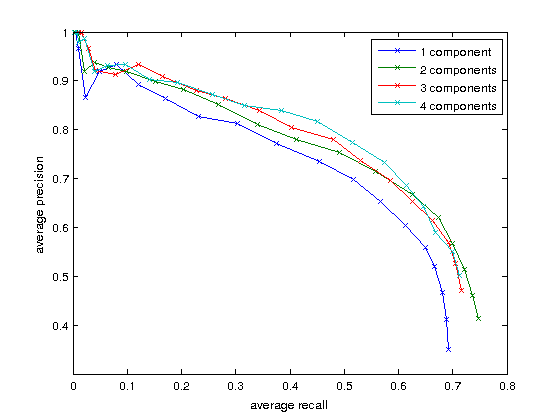Average precision/recall curves across each of the 5 test sets of cross validation by number of components used. The closer to the upper-right, the better. This makes clear that using more than 1 component improve results considerably.

Looking at the results, it is clear that using multiple components - i.e. multiple distinct models instead of just one - improves on the results significantly. This means that animation characters show some large variations which the multiple component model seems to pick up on. Picking a high recall threshold for detection seems to be sensible - we will get more false positives in exchange for more true positives, which is an acceptable trade-off for web artist communities. This gets us a recall of roughly 70% using a multi-components model, which means that a given character in an image has a 70% chance of being detected by our algorithm.

## What’s next?

Now that I have a satisfying method for character detection, I would like to focus on identifying the detected character. For this, I am currently designing a method based on the deformable parts model also used for Felzenszwalb’s detection method. If everything goes well - i.e. if I can implement and test it in time - this will be the subject of a paper I will submit to the ICISIP 2014 conference. I am planning on submitting it to arXiv around the same time, and this should be the subject of my next post.

## Thanks

I would like to thank my labmate Yuki Nakagawa, who allowed me to use his artwork for my blog posts and papers. Please check his work out on Pixiv :) ! I would like to thank Andreas Mass, Hugo Duprat, Matthias Paillard and Joseph Kochmann for their feedback on the previous post and this one.